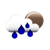# Kilowatt-hour to BTU (kWh to btu)

 Example: 1 kilowatt hour = 3412.14163 btu kWh: kWh BTU: btu
You may also interested in: btu to Kilowatt-hour Converter

The online kWh to btu Converter is used to convert the energy from kWh to btu.

#### The kWh to BTU Conversion Formula

You can use the following formula to convert from kWh to BTU :

X(BTU) = y(kWh) × 3412.14163312794

Example: How to convert 1 kilowatt-hour to BTU ?

X(BTU) = 1(kWh) × 3412.14163312794

#### Kilowatt-hour to BTU conversion table


Kilowatt-hour (kWh) BTU (btu)
1 kWh3412.141633 btu
2 kWh6824.283266 btu
3 kWh10236.424899 btu
4 kWh13648.566533 btu
5 kWh17060.708166 btu
6 kWh20472.849799 btu
7 kWh23884.991432 btu
8 kWh27297.133065 btu
9 kWh30709.274698 btu
10 kWh34121.416331 btu
20 kWh68242.832663 btu
30 kWh102364.248994 btu
40 kWh136485.665325 btu
50 kWh170607.081656 btu
60 kWh204728.497988 btu
70 kWh238849.914319 btu
80 kWh272971.33065 btu
90 kWh307092.746982 btu
100 kWh341214.163313 btu
500 kWh1706070.816564 btu
1000 kWh3412141.633128 btu

To know how to convert kilowatt hours to BTU, please use our kilowatt hours to BTU Converter for free.

#### References

More references for kilowatt hours and BTU

#### Local Weather Status

Ashburn, US
18th January, 2020 Saturday
Moderate Rain-1.11 - 1.67
Humidity: 92 %
Wind: 4.1 km/h